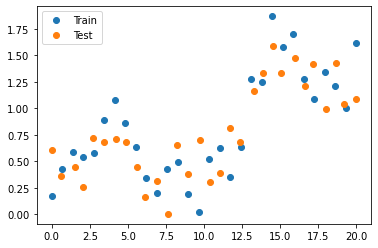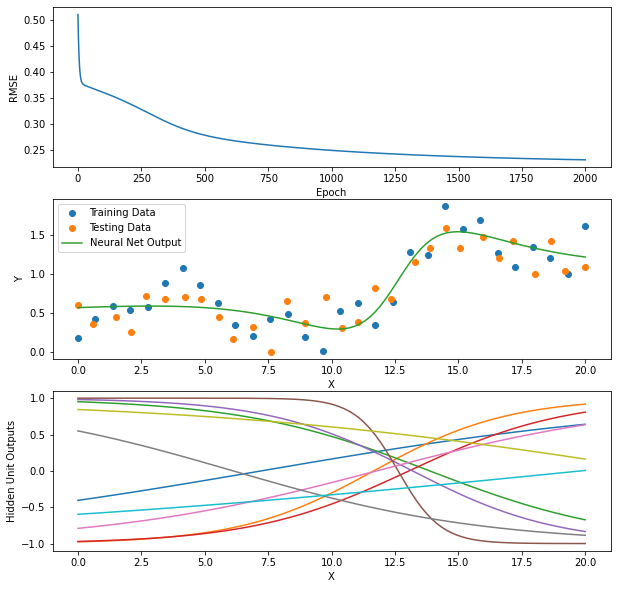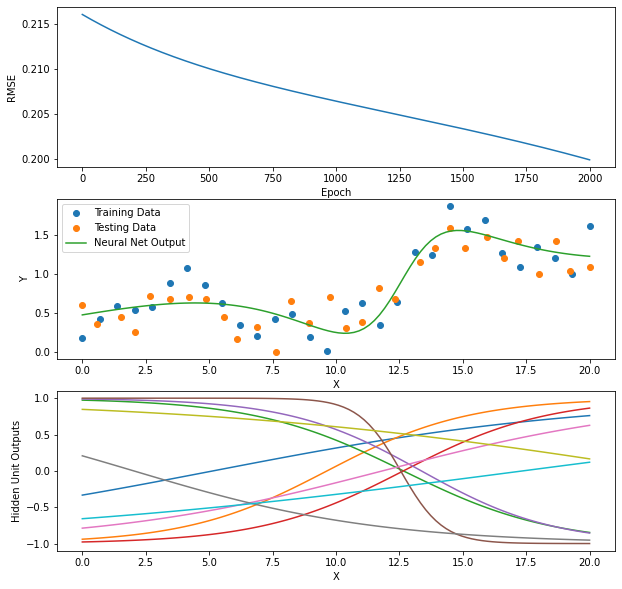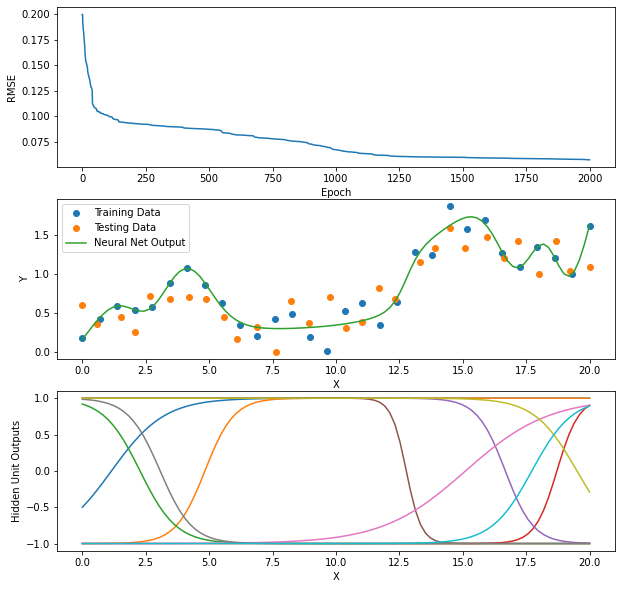# Collect Weights in Vector for Optimizers¶

Do you remember the optimizers.py code we used earlier in the semester? These required all weights to be collected into a flat vector.

Download optimizers.tar and extract optimizers.py.

At the bottom of optimizers.py, in the __main__ section of code, we have these simple tests.

In :
import numpy as np
import matplotlib.pyplot as plt

import optimizers as opt

In :
def parabola(wmin):
return ((w - wmin) ** 2)

return 2 * (w - wmin)

wmin = 5

print()
w = np.array([0.0])
optimizer = opt.Optimizers(w)
print(f'sgd: Minimum of parabola is at {wmin}. Value found is {w}\n')

w = np.array([0.0])
optimizer = opt.Optimizers(w)
print(f'adam: Minimum of parabola is at {wmin}. Value found is {w}\n')

w = np.array([0.0])
optimizer = opt.Optimizers(w)
print(f'scg: Minimum of parabola is at {wmin}. Value found is {w}')

sgd: Epoch 5 Error=4.19430
sgd: Epoch 10 Error=0.45036
sgd: Epoch 15 Error=0.04836
sgd: Epoch 20 Error=0.00519
sgd: Epoch 25 Error=0.00056
sgd: Epoch 30 Error=0.00006
sgd: Epoch 35 Error=0.00001
sgd: Epoch 40 Error=0.00000
sgd: Epoch 45 Error=0.00000
sgd: Epoch 50 Error=0.00000
sgd: Minimum of parabola is at 5. Value found is [4.99992864]

adam: Minimum of parabola is at 5. Value found is [4.0988809]

scg: Minimum of parabola is at 5. Value found is [5.]


Let's see how to use Optimizers for our one-hidden-layer neural network code.

First, here are the standardization functions which we won't need to change.

In :
def calc_standardize_parameters(X, T):
Xmeans = X.mean(axis=0)
Xstds = X.std(axis=0)
Tmeans = T.mean(axis=0)
Tstds = T.std(axis=0)
return {'Xmeans': Xmeans, 'Xstds': Xstds,
'Tmeans': Tmeans, 'Tstds': Tstds}

def standardize_X(X, stand_parms):
return (X - stand_parms['Xmeans']) / stand_parms['Xstds']

def unstandardize_X(Xst, stand_parms):
return Xst * stand_parms['Xstds'] + stand_parms['Xmeans']

def standardize_T(T, stand_parms):
return (T - stand_parms['Tmeans']) / stand_parms['Tstds']

def unstandardize_T(Tst, stand_parms):
return Tst * stand_parms['Tstds'] + stand_parms['Tmeans']


And here are other functions that won't change.

In :
def add_ones(X):
return np.insert(X, 0, 1, axis=1)

def rmse(Y, T):
error = T - Y
return np.sqrt(np.mean(error ** 2))


Now, we need to collect all of the weight values from each layer into one long vector, that we will call all_weights. This will be a global variable that our functions can use. We also define two global variables to be our "views" into all_weights for V and W.

In :
all_weights = None
V = None
W = None

In :
def make_weights(n_inputs, n_hiddens, n_outputs):
# First, let's allocate contiguous memory to hold all weights
n_V = (1 + n_inputs) * n_hiddens
n_W = (n_hiddens + 1) * n_outputs
n_weights = n_V + n_W
all_weights = np.zeros(n_weights)

# Now we create numpy views into this memory with the appropriate shapes to be our weight matrices.
V = all_weights[:n_V].reshape(1 + n_inputs, n_hiddens)
W = all_weights[n_V:].reshape(1 + n_hiddens, n_outputs)

# Initialize each weight matrix to uniformly-distribted random values between -sqrt(n_in) and +sqrt(n_in)
V[:] = np.random.uniform(-1, 1, size=(1 + n_inputs, n_hiddens)) / np.sqrt(n_inputs + 1)
W[:] = np.random.uniform(-1, 1, size=(1 + n_hiddens, n_outputs)) / np.sqrt(n_hiddens + 1)

return all_weights, V, W

In :
all_weights, V, W

Out:
(None, None, None)
In :
all_weights, V, W = make_weights(1, 2, 3)

In :
all_weights, V, W

Out:
(array([ 0.60925941, -0.52591064, -0.27557238,  0.17959754, -0.43340268,
0.2420728 ,  0.54635732, -0.39758013, -0.15481982, -0.17338991,
-0.1583756 , -0.09093871, -0.57640149]),
array([[ 0.60925941, -0.52591064],
[-0.27557238,  0.17959754]]),
array([[-0.43340268,  0.2420728 ,  0.54635732],
[-0.39758013, -0.15481982, -0.17338991],
[-0.1583756 , -0.09093871, -0.57640149]]))
In :
all_weights.shape,  V.shape,  W.shape

Out:
((13,), (2, 2), (3, 3))
In :
V[0, 0]

Out:
0.6092594059348118
In :
V[0, 0]=42
V

Out:
array([[42.        , -0.52591064],
[-0.27557238,  0.17959754]])
In :
all_weights

Out:
array([42.        , -0.52591064, -0.27557238,  0.17959754, -0.43340268,
0.2420728 ,  0.54635732, -0.39758013, -0.15481982, -0.17338991,
-0.1583756 , -0.09093871, -0.57640149])

Now we can modify our other functions to use these global variables.

In :
V[0, 0] = -0.137

In :
# def forward(Xst, V, W):
def forward(Xst):  # ONLY CHANGE NEEDED
# Calculate the outputs, Z, of all hidden units, given all input samples in X.
# Calculate the outputs, Y, of all output units, given all outputs of the hidden units.
return Z, Yst

In :
forward(np.array([-1, 0, 2, 5]).reshape(-1, 1))

Out:
(array([[ 0.13769217, -0.6078524 ],
[-0.13614927, -0.48224896],
[-0.59678883, -0.16518798],
[-0.90779805,  0.35580715]]),
array([[-0.39187736,  0.27603264,  0.87284992],
[-0.30289596,  0.3070065 ,  0.84793325],
[-0.16996955,  0.34948952,  0.74504908],
[-0.12883138,  0.35026129,  0.49867257]]))

Now, for backward, we also need a global variable to hold all of the gradient values for both layers, and our views into this vector for the gradients for weights in each layer. Since the matrix of gradients with respect to the weights in a layer is exactly the same shape as the weight matrix for that layer, we can reuse our make_weights function.

In :
all_gradients, gradient_V, gradient_W = make_weights(1, 2, 3)

In :
all_gradients.shape, all_weights.shape

Out:
((13,), (13,))
In :
gradient_V.shape, V.shape,  gradient_W.shape, W.shape

Out:
((2, 2), (2, 2), (3, 3), (3, 3))
In :
# def backward(Xst, Tst, V, W):
def backward(Xst, Tst):                                    # CHANGED

n_samples = Xst.shape
n_outputs = Tst.shape
# Calculate the outputs of both layers.
Z, Yst = forward(Xst)                           # CHANGED
# Calculate the delta value for the output layer. Divide by n_samples * n_outputs
# because we are calculating the gradient of the mean sqauared error with respect to weights.
delta = -(Tst - Yst) /  (n_samples * n_outputs)
# The gradient of the mean squared error with respect to the output layer weights W.
# Back-propagate the delta value from the output layer, through the output layer weights,
# to the hidden units.  Multiply the result by the derivative of the hidden units'
# activation function, tanh
delta = (delta @ W[1:, :].T) * (1 - Z ** 2)
# The gradient of the mean squared error with respect to the hidden layer weights, V.
# Return both gradients.  Each should be the same shape as the respective weight matrices.

In :
# def train_sgd(X, T, V, W, learning_rate, n_epochs):
def train_sgd(X, T, learning_rate, n_epochs):                           # CHANGED
global V, W

# Store standardization parameters in dictionary stand_parms.
stand_parms = calc_standardize_parameters(X, T)
# Standardize X and T.
Xst = standardize_X(X, stand_parms)
Tst = standardize_T(T, stand_parms)

error_trace = []

# Update weights for n_epochs passes through the training data
for epoch in range(n_epochs):

# Calculate the gradients of the mean squared error with respect to each weight matrix.
backward(Xst, Tst)                                              # CHANGED, Don't need the returned values

# Update the values in each weight matrix using SGD.
V -= learning_rate * gradient_V                                 # DON'T NEED [:] because of -=

# Calculate the outputs of both layers given the current weight values.
_, Yst = forward(Xst)                                           # CHANGED
Y = unstandardize_T(Yst, stand_parms)
error_trace.append(rmse(Y, T))

# return V, W, stand_parms, error_trace
return stand_parms, error_trace                                     # CHANGED

In :
# def use(X, V, W, stand_parms):
def use(X, stand_parms):
# Standardize inputs X
Xst = standardize_X(X, stand_parms)
# Calculate outputs of each layer.
Z, Yst = forward(Xst)                                               # CHANGED
# Unstandardize output of output layer
return Z, unstandardize_T(Yst, stand_parms)


Now we can run our toy data again from A2.

In :
n_samples = 30

Xtrain = np.linspace(0., 20.0, n_samples).reshape((n_samples, 1))
Ttrain = 0.2 + 0.05 * (Xtrain) + 0.4 * np.sin(Xtrain / 2) + 0.2 * np.random.normal(size=(n_samples, 1))

Xtest = Xtrain + 0.1 * np.random.normal(size=(n_samples, 1))
Ttest = 0.2 + 0.05 * (Xtest) + 0.4 * np.sin(Xtest / 2) + 0.2 * np.random.normal(size=(n_samples, 1))

plt.plot(Xtrain, Ttrain, 'o', label='Train')
plt.plot(Xtest, Ttest, 'o', label='Test')
plt.legend();In :
n_inputs = Xtrain.shape
n_hiddens = 10
n_outputs = Ttrain.shape

n_epochs = 2000
learning_rate = 0.1

all_weights, V, W = make_weights(n_inputs, n_hiddens, n_outputs)

stand_parms, error_trace = train_sgd(Xtrain, Ttrain, learning_rate, n_epochs)

_, Ytrain = use(Xtrain, stand_parms)  #
rmse_train = rmse(Ytrain, Ttrain)
_, Ytest = use(Xtest, stand_parms)
rmse_test = rmse(Ytest, Ttest)

print(f'RMSE: Train {rmse_train:.2f} Test {rmse_test:.2f}')

RMSE: Train 0.23 Test 0.20

In :
def show_plots():
plt.figure(figsize=(10, 10))
plt.subplot(3, 1, 1)
plt.plot(error_trace)
plt.xlabel('Epoch')
plt.ylabel('RMSE')

plt.subplot(3, 1, 2)
plt.plot(Xtrain, Ttrain, 'o', label='Training Data')
plt.plot(Xtest, Ttest, 'o', label='Testing Data')
X_for_plot = np.linspace(0, 20, 100).reshape(-1, 1)
Z_train, Y_train = use(X_for_plot, stand_parms)
plt.plot(X_for_plot, Y_train, label='Neural Net Output')
plt.legend()
plt.xlabel('X')
plt.ylabel('Y')

plt.subplot(3, 1, 3)
plt.plot(X_for_plot, Z_train)
plt.xlabel('X')
plt.ylabel('Hidden Unit Outputs')

show_plots()## Again with Optimizers¶

We need to add a function for the quantity we wish to minimze, the mean-squared-error.

In :
def mse(Xst, Tst):
Z, Yst = forward(Xst)
return np.mean((Tst - Yst)**2)

In :
def train(X, T, learning_rate, n_epochs, method='sgd'):
global V, W

# Store standardization parameters in dictionary stand_parms.
stand_parms = calc_standardize_parameters(X, T)
# Standardize X and T.
Xst = standardize_X(X, stand_parms)
Tst = standardize_T(T, stand_parms)

optimizer = opt.Optimizers(all_weights)

if method == 'sgd':
error_trace = optimizer.sgd(mse, backward, [Xst, Tst], learning_rate, n_epochs)
error_trace = optimizer.adam(mse, backward, [Xst, Tst], learning_rate, n_epochs)
elif method == 'scg':
error_trace = optimizer.scg(mse, backward, [Xst, Tst], learning_rate, n_epochs)
else:
print('method must be ''sgd'', ''adam'', or ''scg''.')

return stand_parms, error_trace

In :
stand_parms, error_trace = train(Xtrain, Ttrain, learning_rate, n_epochs)

_, Ytrain = use(Xtrain, stand_parms)  #
rmse_train = rmse(Ytrain, Ttrain)
_, Ytest = use(Xtest, stand_parms)
rmse_test = rmse(Ytest, Ttest)

print(f'RMSE: Train {rmse_train:.2f} Test {rmse_test:.2f}')

sgd: Epoch 200 Error=0.21323
sgd: Epoch 400 Error=0.21100
sgd: Epoch 600 Error=0.20923
sgd: Epoch 800 Error=0.20775
sgd: Epoch 1000 Error=0.20644
sgd: Epoch 1200 Error=0.20520
sgd: Epoch 1400 Error=0.20398
sgd: Epoch 1600 Error=0.20271
sgd: Epoch 1800 Error=0.20136
sgd: Epoch 2000 Error=0.19988
RMSE: Train 0.22 Test 0.21

In :
show_plots()In :
stand_parms, error_trace = train(Xtrain, Ttrain, learning_rate, n_epochs, method='scg')

_, Ytrain = use(Xtrain, stand_parms)  #
rmse_train = rmse(Ytrain, Ttrain)
_, Ytest = use(Xtest, stand_parms)
rmse_test = rmse(Ytest, Ttest)

print(f'RMSE: Train {rmse_train:.2f} Test {rmse_test:.2f}')

SCG: Epoch 200 Error=0.19987
SCG: Epoch 400 Error=0.19987
SCG: Epoch 600 Error=0.19987
SCG: Epoch 800 Error=0.19987
SCG: Epoch 1000 Error=0.19987
SCG: Epoch 1200 Error=0.19987
SCG: Epoch 1400 Error=0.19987
SCG: Epoch 1600 Error=0.19987
SCG: Epoch 1800 Error=0.19987
SCG: Epoch 2000 Error=0.19987
RMSE: Train 0.12 Test 0.25

In :
show_plots()